# Sorting in the Bit Model, Lower Bounds

Tom Kelliher, CS 315

Feb. 3, 1999

### From Last Time

Sorting in the Bit Model.

### Outline

1. Sorting in the bit model.

2. Example problems.

3. Lower bound techniques.

### Coming Up

Lower bound counterexample, properties of fixed-connection networks.

# Sorting in the Bit Model

1. Why get more detailed? To see how much work (e.g., #number of transistors) is involved in, for instance, comparison.

2. A linear array bit-level model of a compare cell: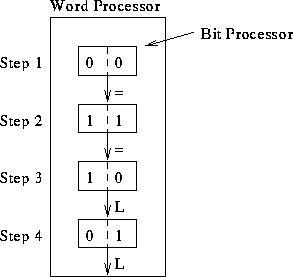Compare, notify.

Assume we have k-bit numbers. Run time?

3. Can we do better? Yes. We can do comparisons in parallel and merge the partial results: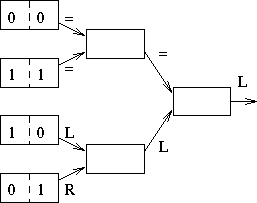Compare, notify.

Run time?

Notes:

1. Why not use a height 1 tree?

2. Fan-in and fan-out in circuits.

## Sorting

1. Run-time for sorting on a linear array of linear array comparators. Binary tree comparators.

What do they look like?

2. Pipelining the linear comparators. Pipelining the compare: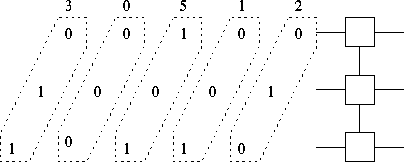Five in, four out.

A lot of parallel work. Systolic computation.

3. Pipelining the sort. Just a linear array of the comparators:Running time?

# Example Problems

1.2
Consider two algorithms for solving a a problem of size M, one that runs in M steps on an M-processor machine and one that runs in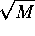steps on an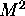-processor machine. Which algorithm is more efficient?

Assume that the cost of running a P-processor machine for T steps is, where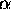and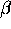are constants and P = M or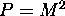. For what values ofandis the first algorithm cheaper to run? For what values ofandis the second algorithm cheaper to run?

1.3
Consider two algorithms for solving a problem of size M, one that runs in M steps on an M-processor machine and one that runs insteps on an-processor machine. Which algorithm will run faster on an N-processor machine? (Hint: Your answer depends on the relative sizes of M and N, and you will need to use the fact that an N-processor machine can simulate a P-processor machine with slowdown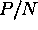.)

# Lower Bound Arguments

The following are tools we can sometimes use to characterize a problem-solving network and, hence, understand its failings.

Assume the bit model, so we have an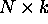sorting network in the following.

1. I/O bandwidth.

What is the I/O bandwidth of the sorting network? This makes sorting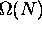.

2. Diameter of the network: Defined as the maximum distance between two vertices in the graph.

What is the diameter of the sorting graph?

Can we construct a sorting problem that will force the two most distant cells to communicate?

What's the lower bound from this perspective?

3. Bisection width of the network: Defined as the number of edges which must be cut to split the graph in ``half.''

What is the bisection width of the sorting graph?

Can we construct a sorting problem that will force all the data to cross this boundary?

What's the lower bound from this perspective?

Thomas P. Kelliher
Wed Feb 3 08:18:46 EST 1999
Tom Kelliher